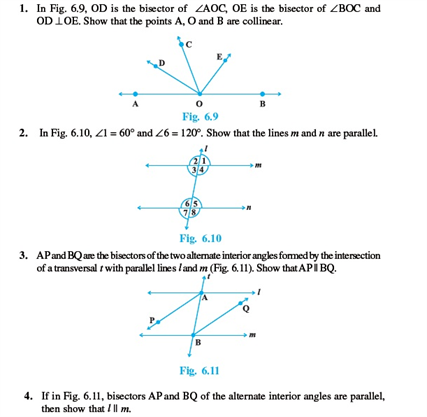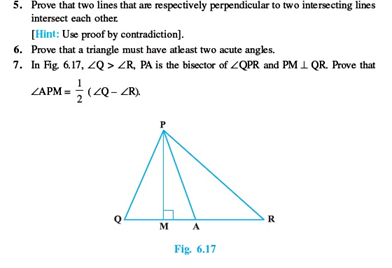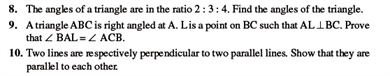# Revision Notes For CBSE Class 9 Math Chapter -6 Lines and Angles

Lines and Angles are the two basic elements of geometry. If the lines or line segments intersect, then the angles are formed.

Lines are the straight figures, made up of infinite points, extending infinitely in both the directions. They have negligible depth or width. There are four types of lines: horizontal line, vertical line, perpendicular, and parallel lines.

An angle is a figure formed from the two rays emerging from a common endpoint.

Listed below are types of angles:

• Acute angle – 0 to 90 degrees.
• Right angle- 90-degree angle.
• Obtuse angle- 90 to 180 degrees.
• Straight angle- 180-degree angle.

Check here for the free CBSE Class 9 Math revision notes for Chapter 6- Lines and Angles